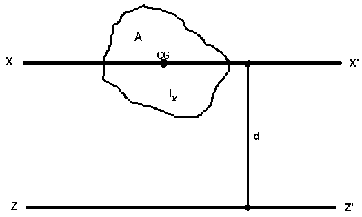Parallel axis theoremBy Wikipedia,
the free encyclopedia,

http://en.wikipedia.org/wiki/Parallel_axes_rule

In physics, the parallel axis theorem or Steiner's theorem can be used to determine the moment of inertia of a rigid body about any axis, given the moment of inertia of the object about the parallel axis through the object's center of mass and the perpendicular distance between the axes.

Let:
Icm denote the moment of inertia of the object about its centre of mass,
M the object's mass and d the perpendicular distance between the two axes.

Then the moment of inertia about the new axis z is given by:$I_z = I_{cm} + Md^2.\,$

This rule can be applied with the stretch rule and perpendicular axis theorem to find moments of inertia for a variety of shapes.Parallel axes rule for area moment of inertia.

The parallel axes rule also applies to the second moment of area (area moment of inertia) for a plane region D:$I_z = I_x + Ad^2.\,$

In this formula,
Iz is the area moment of inertia of D relative to the parallel axis,
Ix is the area moment of inertia of D relative to its centroid,
A is the area of the plane region D, and
d is the distance from the new axis z to the centroid of the plane region D.
Note: The centroid of D coincides with the center of gravity (CG) of a physical plate with the same shape that has constant density.

The parallel axis theorem is one of several theorems referred to as Steiner's theorem, after Jakob Steiner.

## In classical mechanics

In classical mechanics, the Parallel axis theorem (also known as Huygens-Steiner theorem) can be generalized to calculate a new inertia tensor Jij from an inertia tensor about a center of mass Iij when the pivot point is a displacement a from the center of mass:

Jij = Iij + M(aδijaiaj)

where$\boldsymbol{a}=a_1\boldsymbol{\hat{x}}+a_2\boldsymbol{\hat{y}}+a_3\boldsymbol{\hat{z}}$

is the displacement vector from the center of mass to the new axis, and

δij

is the Kronecker delta.

We can see that, for diagonal elements (when i = j), displacements perpendicular to the axis of rotation results in the above simplified version of the parallel axis theorem.

## See also

Text from Wikipedia is available under the Creative Commons Attribution/Share-Alike License; additional terms may apply.

Published - July 2009

Please see some ads intermixed with other content from this site:Copyright 2004-2021 © by Airports-Worldwide.com, Vyshenskoho st. 36, Lviv 79010, Ukraine
Legal Disclaimer